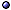In General > s.a. history of mathematics.
* Idea: The study of patterns; It arises from interaction of empirical facts and abstract ideas.
* Debates: (a) Pure vs applied mathematics, what is what? (b) Does mathematics create or discover? (See positions of Cantor and Kronecker, respectively); For the second point of view, see Chern on fiber bundles [@ in Yang PNYAS(77)].
* And reasoning: Reasoning is usually based on logic, but one can shift the emphasis to combinatorics.
* Interconnections: Examples are algebraic topology, and the Langlands program.

Major Programs, Problems, Areas > s.a. conjectures; mathematical physics.
* Hilbert's Program, in general: In 1900, Hilbert proposed 23 problems that seemed to him to be the most difficult and rewarding ones in mathematics; 2000, Three of them are not yet fully solved, and one of them, the Riemann Hypothesis, is a completely open question.
* Hilbert's 6th Problem: "To treat by means of axioms, those physical sciences in which mathematics plays an important part."
* Langlands Program: A vast mathematical vision formulated by Robert Langlands to unite whole areas of mathematics; The theory of automorphic forms and its connection with L-functions and other fields; A special case is the Shimura-Taniyama-Weil conjecture.
* 2000: The Clay Mathematics Institute has offered a new list of seven outstanding Millennium Problems (including the Riemann Hypothesis, Poincaré Conjecture, P vs NP Problem, and Navier-Stokes Equation, whether the equations develop singularities), offering \$1M for a verified solution to each.
@ General references: Glimm BAMS(10) [challenges and opportunities]; Wolpert & Kinney a2012-FQXi [mathematics as a fundamentally stochastic process].
@ Hilbert's Program: Hilbert MN(1900), BAMS(02), reprinted BAMS(00); Kantor MI(96) [status], Ilyashenko BAMS(02) [16th]; Gray 00; Yandell 02 [problems and solvers]; news sn(19)nov [10th problem, contribution by Julia Robinson].
@ Hilbert's Program, 6th Problem: Dass a0909, IJNS?-a1002, Pra(11)-a1006; Schreiber a1311-proc [classical field theory from cohesive homotopy type theory]; Gorban PTRS(18)-a1803 [intro].
@ Langlands Program: Frenkel BAMS(04); Frenkel ht/05-ln [and quantum field theory]; Frenkel a0906-talk [geometric, and gauge theory]; Frenkel BAMS(13) [trace formulas and geometrization].
@ Millennium Problems: Smith m.DG/06-wd [Navier-Stokes claim, withdrawn]; Chatterjee JFA-a1602 [Yang-Mills free energy]; Jaffe & Xue a2007 [little-known anecdotes]; > s.a. CMI page.Main areas: see algebra; analysis; Arithmetic; combinatorics; differential equations; geometry; logic; number theory; probability; set theory; topology.More specific topics: see inequalities; Mathematical Constants; matrices; proof theory; Relations; series; Solvability.

Foundations > s.a. numbers [rational, real].
* Peano's axioms:
(i) For all x in $$\mathbb N$$, 0 ≠ x + 1;
(ii) For all x, y in $$\mathbb N$$, x + 1 = y + 1 only if x = y;
(iii) MN, and M ≠ Ø implies that M has a smallest element with respect to <;
(iv) For all x, y in $$\mathbb N$$, xy precisely when there exists z in $$\mathbb N$$ such that x + z = y;
(v) The operations + and · satisfy, for all x, y in $$\mathbb N$$: x + (y+1) = (x+y) + 1; x + 0 = x; x · (y+1) = x · y + x; x · 0 = 0.
@ References: Engeler 93 [short]; Chaitin AS(02) [randomness and paradoxes], SA(06)mar [limitations].

Philosophy
* Intuitionism: "Intuitionism seeks to break up and to disfigure mathematics" [@ Hilbert 35, p188]; > s.a. time [and physics]; Wikipedia page.
* "The universe of mathematics grows out of the world about us like dreams out of the events of the day" [@ Stein 69].
@ References: in Wigner CPAM(60); Hersh AiM(79); Field 89; Maddy 93 [realism]; Brown 99; Shapiro 00, 00, 05; Linnebo & Uzquiano BJPS(09) [acceptable abstraction principles]; Baker BJPS(09) [explanations in science and existence of mathematical entities]; Cellucci SHPSA(13); Werndl PhM-a1310 [justifying definitions].

General References
@ Books: Courant & Robbins 41; Pólya 62, 68; Bochner 66; Saaty & Weyl ed-69; Stein 69; Iyanaga & Kawada 80; Kramer 81; Campbell & Higgins ed-84; Dunham 90; Bajnok 13 [abstract mathematics, II]; Kohar 16 [discrete mathematics].
@ Method: Pólya 57; Van Gasteren 90; Tao BAMS(07) ["good mathematics"]; Kjeldsen & Carter SHPSA(12) [growth of mathematical knowledge]; Roytvarf 13; Mazur 15 [history of mathematical notation].
@ I, books: Jones 70 [non-standard]; Davis & Hersch 81; Dieudonné 92; Casti 95; Devlin 94, 99, 00; Joyner 08 [toys]; Gallier 11 [discrete mathematics]; Herrmann 12.
@ I, short topics: Honsberger 73, 76; Newman 82; Davis & Chinn 85; Ekeland 88; Peterson 88, 90; Barrow 92; Stewart 92; Devlin 03 [unsolved problems]; Tubbs 08; Havil 10 [paradoxes]; Stewart 12 [17 equations that changed the world]; Mackenzie 12 [the story of mathematics in 24 equations]; Beardon 16.
@ Reference books: Smith 59; Hazewinkel 8700.
@ Doing mathematics: Krieger 15; Meier & Smith 17 [and proofs].
@ Conceptual: Friedman & Flagg AAM(90) [complexity of mathematical concepts]; Lucas 99; Rotman 00; Sherry SHPSA(06) [mathematical reasoning]; Josephson a1307-in [is mathematical truth a human construct?]; Tallant BJPS(13) [pretense theories of mathematics fail]; Lev PPNL(17)-a1409 [standard vs finite mathematics]; Dantas ch(16)-a1506-FQXi [as tactics of self-referential systems]; > s.a. Meaning.

Teaching, Applications, and Related Topics > s.a. Machine Learning.
@ General references: Kline 59; Casti 89 [models of nature]; Schwarz PhSc(95)jun [psychology]; Hersh 97; Zebrowski 99 [and the physical universe]; Rotman 00 [as an activity]; Lakoff & Núñez 00 [and cognitive science]; Arianrhod 05 [as language]; Deem PT(07)jan [biology].
@ Teaching: Pólya 57; Wickelgren 74; Krantz 94; Burton 04 [learning]; Bass BAMS(05) [mathematicians and math education]; Hewson 09 [bridge to university-level mathematics]; Li 11 [problems]; Hiriart-Urruty 16 [non-standard exercises].
@ Innumeracy: Paulos 90; NS(91)mar30, p44 [need for mathematics].
@ Quotations: Gaither & Cavazos-Gaither 98.
@ Related topics: Hellman; Knuth BAMS(79) [typography]; Ruelle BAMS(88); Gardner SA(98)aug [recreational]; Renteln & Dundes NAMS(05) [humor]; Silva et al JPA(10) [network of mathematical knowledge]; news nat(11)jul + plus(11)jul [unplanned impact].

Online Resources
> Encyclopedias: see MathPages; MathWorld; Internet Encyclopedia of Science; PlanetMath.org; Platonic Realms; Springer Online Encyclopaedia of Mathematics.
> Other resources: see Earliest Use of Math Terms; MathSciNet [reviews].

"In mathematics the art of proposing a question must be held of higher value than solving it,"
Georg Cantor (1845 – 1918)